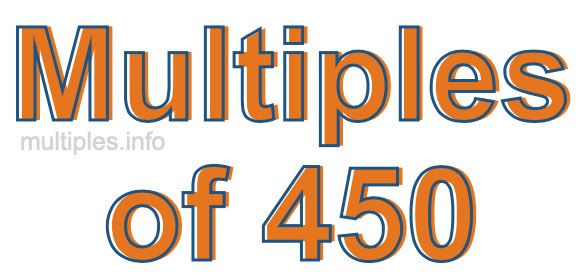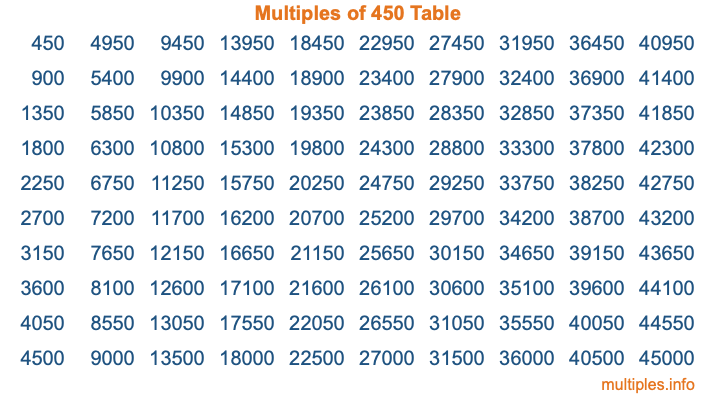Multiples of 450Welcome to the Multiples of 450 page. Here we will first teach you everything you will ever need to know about the multiples of 450, and then give you a study guide summary of everything we taught you to make sure you remember it all. Use this page to look up facts and learn information about the multiples of 450. This page will make you a multiples of four hundred fifty expert!

Definition of Multiples of 450
Multiples of 450 are all the numbers that when divided by 450 equal an integer. Each of the multiples of 450 are called a multiple. A multiple of 450 is created by multiplying 450 by an integer.

Therefore, to create a list of multiples of 450, you start with 1 multiplied by 450, then 2 multiplied by 450, then 3 multiplied by 450, and so on for as long as you want. Thus, the list of the first five multiples of 450 is 450, 900, 1350, 1800, and 2250. To see a larger list of multiples of 450, see the printable image of Multiples of 450 further down on this page. We also have a category where you can choose any nth multiple of 450.

Multiples of 450 Checker
The Multiples of 450 Checker below checks to see if any number of your choice is a multiple of 450. In other words, it checks to see if there is any number (integer) that when multiplied by 450 will equal your number. To do that, we divide your number by 450. If the the quotient is an integer, then your number is a multiple of 450.

Is  a multiple of 450?

Least Common Multiple of 450 and ...
A Least Common Multiple (LCM) is the lowest multiple that two or more numbers have in common. This is also called the smallest common multiple or lowest common multiple and is useful to know when you are adding our subtracting fractions. Enter one or more numbers below (450 is already entered) to find the LCM.

Check out our LCM Calculator if you need more details about the Least Common Multiple or if you need the LCM for different numbers for adding and subtraction fractions.

nth Multiple of 450
As we stated above, 450 is the first multiple of 450, 900 is the second multiple of 450, 1350 is the third multiple of 450, and so on. Enter a number below to find the nth multiple of 450.

th multiple of 450

Multiples of 450 vs Factors of 450
450 is a multiple of 450 and a factor of 450, but that is where the similarities end. All postive multiples of 450 are 450 or greater than 450. All positive factors of 450 are 450 or less than 450.

Below is the beginning list of multiples of 450 and the factors of 450 so you can compare:

Multiples of 450: 450, 900, 1350, 1800, 2250, etc.

Factors of 450: 1, 2, 3, 5, 6, 9, 10, 15, 18, 25, 30, 45, 50, 75, 90, 150, 225, 450

As you can see, the multiples of 450 are all the numbers that you can divide by 450 to get a whole number. The factors of 450, on the other hand, are all the whole numbers that you can multiply by another whole number to get 450.

It's also interesting to note that if a number (x) is a factor of 450, then 450 will also be a multiple of that number (x).

Multiples of 450 vs Divisors of 450
The divisors of 450 are all the integers that 450 can be divided by evenly. Below is a list of the divisors of 450.

Divisors of 450: 1, 2, 3, 5, 6, 9, 10, 15, 18, 25, 30, 45, 50, 75, 90, 150, 225, 450

The interesting thing to note here is that if you take any multiple of 450 and divide it by a divisor of 450, you will see that the quotient is an integer.

Multiples of 450 Table
Below is an image of the first 100 multiples of 450 in a table. The table is in chronological order, column by column. The first column has the first ten multiples of 450, the second column has the next ten multiples of 450, and so on.The Multiples of 450 Table is also referred to as the 450 Times Table or Times Table of 450. You are welcome to print out our table for your studies.

Negative Multiples of 450
Although not often discussed or needed in math, it is worth mentioning that you can make a list of negative multiples of 450 by multiplying 450 by -1, then by -2, then by -3, and so on, to get the following list of negative multiples of 450:

-450, -900, -1350, -1800, -2250, etc.

Multiples of 450 Summary
Below is a summary of important Multiples of 450 facts that we have discussed on this page. To retain the knowledge on this page, we recommend that you read through the summary and explain to yourself or a study partner why they hold true.

There are an infinite number of multiples of 450.

A multiple of 450 divided by 450 will equal a whole number.

450 divided by a factor of 450 equals a divisor of 450.

The nth multiple of 450 is n times 450.

The largest factor of 450 is equal to the first positive multiple of 450.

450 is a multiple of every factor of 450.

450 is a multiple of 450.

A multiple of 450 divided by a divisor of 450 equals an integer.

450 divided by a divisor of 450 equals a factor of 450.

Any integer times 450 will equal a multiple of 450.

Multiples of a Number
Here you can get the multiples of another number, all with the same attention to detail as we did for multiples of 450 on this page.

Multiples of
Multiples of 451
Did you find our page about multiples of four hundred fifty educational? Do you want more knowledge? Check out the multiples of the next number on our list!